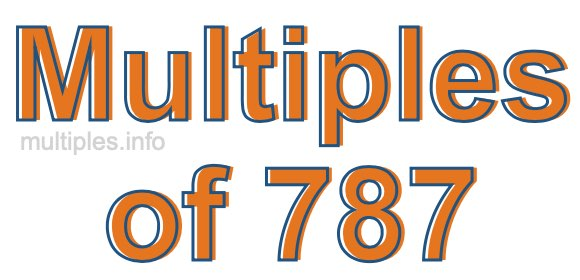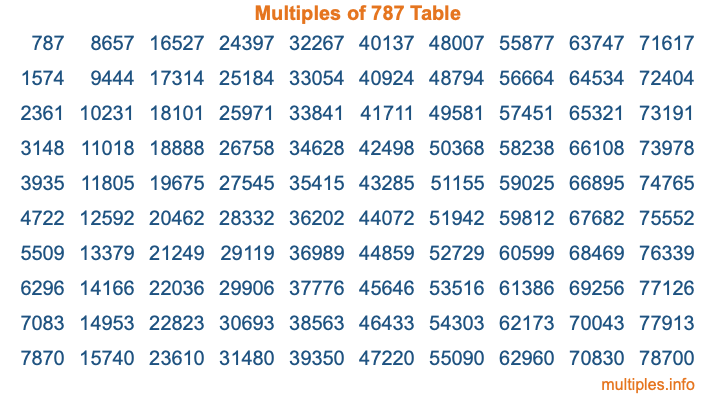Multiples of 787Welcome to the Multiples of 787 page. Here we will first teach you everything you will ever need to know about the multiples of 787, and then give you a study guide summary of everything we taught you to make sure you remember it all. Use this page to look up facts and learn information about the multiples of 787. This page will make you a multiples of seven hundred eighty-seven expert!

Definition of Multiples of 787
Multiples of 787 are all the numbers that when divided by 787 equal an integer. Each of the multiples of 787 are called a multiple. A multiple of 787 is created by multiplying 787 by an integer.

Therefore, to create a list of multiples of 787, you start with 1 multiplied by 787, then 2 multiplied by 787, then 3 multiplied by 787, and so on for as long as you want. Thus, the list of the first five multiples of 787 is 787, 1574, 2361, 3148, and 3935. To see a larger list of multiples of 787, see the printable image of Multiples of 787 further down on this page. We also have a category where you can choose any nth multiple of 787.

Multiples of 787 Checker
The Multiples of 787 Checker below checks to see if any number of your choice is a multiple of 787. In other words, it checks to see if there is any number (integer) that when multiplied by 787 will equal your number. To do that, we divide your number by 787. If the the quotient is an integer, then your number is a multiple of 787.

Is  a multiple of 787?

Least Common Multiple of 787 and ...
A Least Common Multiple (LCM) is the lowest multiple that two or more numbers have in common. This is also called the smallest common multiple or lowest common multiple and is useful to know when you are adding our subtracting fractions. Enter one or more numbers below (787 is already entered) to find the LCM.

Check out our LCM Calculator if you need more details about the Least Common Multiple or if you need the LCM for different numbers for adding and subtraction fractions.

nth Multiple of 787
As we stated above, 787 is the first multiple of 787, 1574 is the second multiple of 787, 2361 is the third multiple of 787, and so on. Enter a number below to find the nth multiple of 787.

th multiple of 787

Multiples of 787 vs Factors of 787
787 is a multiple of 787 and a factor of 787, but that is where the similarities end. All postive multiples of 787 are 787 or greater than 787. All positive factors of 787 are 787 or less than 787.

Below is the beginning list of multiples of 787 and the factors of 787 so you can compare:

Multiples of 787: 787, 1574, 2361, 3148, 3935, etc.

Factors of 787: 1, 787

As you can see, the multiples of 787 are all the numbers that you can divide by 787 to get a whole number. The factors of 787, on the other hand, are all the whole numbers that you can multiply by another whole number to get 787.

It's also interesting to note that if a number (x) is a factor of 787, then 787 will also be a multiple of that number (x).

Multiples of 787 vs Divisors of 787
The divisors of 787 are all the integers that 787 can be divided by evenly. Below is a list of the divisors of 787.

Divisors of 787: 1, 787

The interesting thing to note here is that if you take any multiple of 787 and divide it by a divisor of 787, you will see that the quotient is an integer.

Multiples of 787 Table
Below is an image of the first 100 multiples of 787 in a table. The table is in chronological order, column by column. The first column has the first ten multiples of 787, the second column has the next ten multiples of 787, and so on.The Multiples of 787 Table is also referred to as the 787 Times Table or Times Table of 787. You are welcome to print out our table for your studies.

Negative Multiples of 787
Although not often discussed or needed in math, it is worth mentioning that you can make a list of negative multiples of 787 by multiplying 787 by -1, then by -2, then by -3, and so on, to get the following list of negative multiples of 787:

-787, -1574, -2361, -3148, -3935, etc.

Multiples of 787 Summary
Below is a summary of important Multiples of 787 facts that we have discussed on this page. To retain the knowledge on this page, we recommend that you read through the summary and explain to yourself or a study partner why they hold true.

There are an infinite number of multiples of 787.

A multiple of 787 divided by 787 will equal a whole number.

787 divided by a factor of 787 equals a divisor of 787.

The nth multiple of 787 is n times 787.

The largest factor of 787 is equal to the first positive multiple of 787.

787 is a multiple of every factor of 787.

787 is a multiple of 787.

A multiple of 787 divided by a divisor of 787 equals an integer.

787 divided by a divisor of 787 equals a factor of 787.

Any integer times 787 will equal a multiple of 787.

Multiples of a Number
Here you can get the multiples of another number, all with the same attention to detail as we did for multiples of 787 on this page.

Multiples of
Multiples of 788
Did you find our page about multiples of seven hundred eighty-seven educational? Do you want more knowledge? Check out the multiples of the next number on our list!